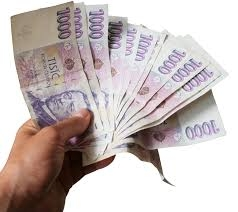# One-fifth 21003

John, Paul, Peter, and Jan earned 42 thousand. John and Paul have the same. Peter is 20% more than Paul. George was one-fifth less than Paul. How much did everyone earn?

A =  10500
I =  8400
J =  10500
P =  12600

### Step-by-step explanation:

J+A+P+I=42000
J=A
P=1.2•A
I=A-A/5

A+I+J+P = 42000
A-J = 0
1.2A-P = 0
4A-5I = 0

A = 10500
I = 8400
J = 10500
P = 12600

Our linear equations calculator calculates it.Did you find an error or inaccuracy? Feel free to write us. Thank you!

Tips for related online calculators